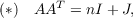#### Vol. 17, No. 1, 1966

 Download this articleFor screen For printingRecent Issues Vol. 325: 1  2 Vol. 324: 1  2 Vol. 323: 1  2 Vol. 322: 1  2 Vol. 321: 1  2 Vol. 320: 1  2 Vol. 319: 1  2 Vol. 318: 1  2Online Archive Volume: Issue:The Journal Subscriptions Editorial Board Officers Contacts Submission Guidelines Submission Form Policies for Authors ISSN: 1945-5844 (e-only) ISSN: 0030-8730 (print) Special Issues Author Index To Appear Other MSP Journals
Integral solutions to the incidence equation for finite projective plane cases of orders n 2 (mod 4)

### Eugene Carlyle Johnsen

Vol. 17 (1966), No. 1, 97–120
##### Abstract

A finite projective plane of order n 2 can be considered as a v,k,λdesign where v = n2 + n + 1, k = n + 1, and λ = 1. As such, it can be characterized by its point-line 0,1 incidence matrix A of order v satisfying the incidence equationwhere J is the matrix of order v consisting entirely of 1’s. Thus, if a plane of order n exists then () has an integral solution A. Ryser has shown that if A is a normal integral solution to () or if A is merely an integral solution to () where n is odd, then A can be made into an incidence matrix for a plane of order n by suitably multiplying its columns by 1. Such an integral solution to () we shall call a type I solution. When A is merely an integral solution to () where n is even, then A may be a type I solution but may also be not of this type. These latter integral solutions to () we shall call type II solutions. Ryser has constructed type II solutions for n = 2 and for all n 0 (mod 4) for which there exists a Hadamard matrix of order n, and Hall and Ryser have constructed a type II solution for n = 10. In this paper we construct type II solutions for some infinite classes of values of n 2 (mod 4). Basic to these constructions is a special class of v,k,λdesigns called skew-Hadamard designs whose incidence matrices form a part of the substructure of our type II solutions. We exhibit examples for n = 26 and 50 and also derive examples for n = 10 and 18.

Primary: 05.27
Secondary: 50.70
##### Milestones